# Shadow of building footprint height

2888
15
09-08-2016 05:07 AMNew Contributor II

I'm interested in calculating the shadow of building footprints, considering the height of buildings, in a certain time and date of the year. I have an attribute including building footprint and height of each of them (attached). I've tried 'sun shadow' tool. The results are not convincing. The shadow volume falls below the surface terrain which is obviously strange! Have a look: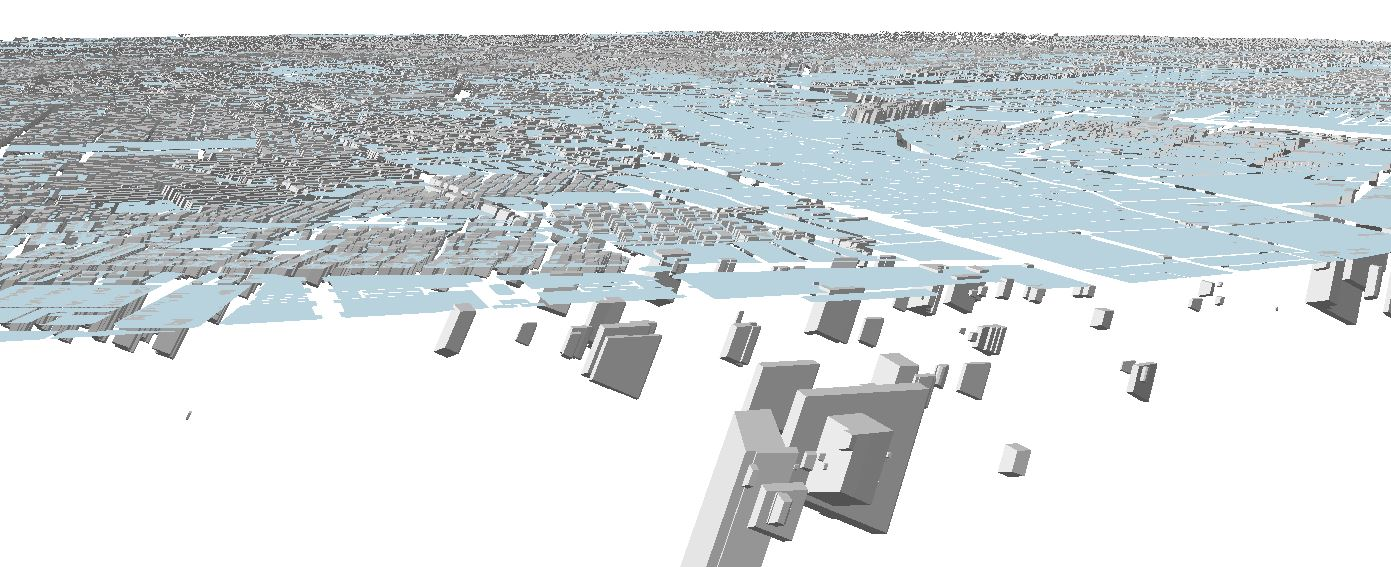Exploring the web I came across a script posted on Geographic Information Systems Stack Exchange. It almost looks like what I would be interested to do. There is a script where one could calculate the 2D shadow of buildings considering their height, however, not specified for a certain time or date of the year.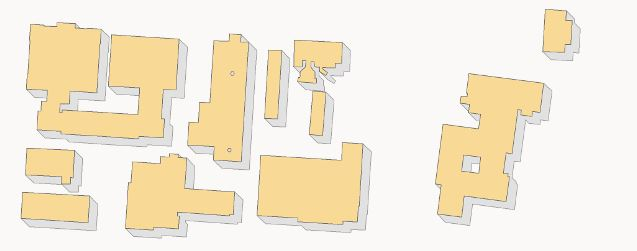here is the link to the web and the script:

``````import arcpy
import os
import math
import multiprocessing
import time

############################    Configuration:    ##############################
# Specify scratch workspace
scratchws = r"c:\temp\bldgshadowtest" # MUST be a folder, not a geodatabase!

# Specify output field name for the original FID
origfidfield = "ORIG_FID"

# Specify the number of processors (CPU cores) to use (0 to use all available)
cores = 0

# Specify per-process feature count limit, tune for optimal
# performance/memory utilization (0 for input row count divided by cores)
procfeaturelimit = 0

# TIP: Set 'cores' to 1 and 'procfeaturelimit' to 0 to avoid partitioning and
# multiprocessing completely
################################################################################

def message(msg, severity=0):
print msg

try:
for string in msg.split('\n'):
if severity == 0:
elif severity == 1:
elif severity == 2:
except:
pass

def getOidRanges(inputFC, oidfield, count):
oidranges = []
if procfeaturelimit > 0:
message("Partitioning row ID ranges ...")
rows = arcpy.SearchCursor(inputFC, "", "", oidfield, "%s A" % oidfield)
minoid = -1
maxoid = -1
for r, row in enumerate(rows):
interval = r % procfeaturelimit
if minoid < 0 and (interval == 0 or r == count - 1):
minoid = row.getValue(oidfield)
if maxoid < 0 and (interval == procfeaturelimit - 1 or r == count - 1):
maxoid = row.getValue(oidfield)
if minoid >= 0 and maxoid >= 0:
oidranges.append([minoid, maxoid])
minoid = -1
maxoid = -1
del row, rows
return oidranges

def computeShadows(inputFC, outputFC, oidfield, shapefield, heightfield, azimuth, altitude, outputSR="", whereclause=""):
# Set outputs to be overwritten just in case; each subprocess gets its own environment settings
arcpy.env.overwriteOutput=True

# Create in-memory feature class for holding the shadow polygons
arcpy.CreateFeatureclass_management(
"in_memory",
"POLYGON", "", "", "",
outputSR)

# Open a cursor on the input feature class
searchfields = ",".join([heightfield, oidfield, shapefield])
rows = arcpy.SearchCursor(inputFC, whereclause, "", searchfields)

# Open an insert cursor on the in-memory feature class

# Create array for holding shadow polygon vertices
arr = arcpy.Array()

for row in rows:
oid = int(row.getValue(oidfield))
shape = row.getValue(shapefield)
height = float(row.getValue(heightfield))

x = -height * spread * math.sin(azimuth)
y = -height * spread * math.cos(azimuth)

# Copy the original shape as a new feature
tempnewrow = tempinscur.newRow()
tempnewrow.setValue(origfidfield, oid) # Copy the original FID value to the new feature
tempnewrow.shape = shape
tempinscur.insertRow(tempnewrow)

# Compute the wall shadow polygons and insert them into the in-memory feature class
for part in shape:
for i,j in enumerate(range(1,part.count)):
pnt0 = part
pnt1 = part
if pnt0 is None or pnt1 is None:
continue # skip null points so that inner wall shadows can also be computed

# Compute the shadow offset points
pnt0offset = arcpy.Point(pnt0.X+x,pnt0.Y+y)
pnt1offset = arcpy.Point(pnt1.X+x,pnt1.Y+y)

# Construct the shadow polygon and insert it to the in-memory feature class
tempnewrow.shape = arr
tempnewrow.setValue(origfidfield, oid) # Copy the original FID value to the new feature
tempinscur.insertRow(tempnewrow)
arr.removeAll() # Clear the array so it can be reused

# Clean up the insert cursor
del tempnewrow, tempinscur

# Dissolve the shadow polygons to the output feature class

# Clean up the in-memory workspace
arcpy.Delete_management("in_memory")

return dissolved

if __name__ == "__main__":
arcpy.env.overwriteOutput=True

inputFC = arcpy.GetParameterAsText(0)
outputFC = arcpy.GetParameterAsText(1)
heightfield = arcpy.GetParameterAsText(2) #Must be in the same units as the coordinate system!
azimuth = math.radians(float(arcpy.GetParameterAsText(3))) #Must be in degrees
altitude = math.radians(float(arcpy.GetParameterAsText(4))) #Must be in degrees

# Get field names
desc = arcpy.Describe(inputFC)
shapefield = desc.shapeFieldName
oidfield = desc.oidFieldName

count = int(arcpy.GetCount_management(inputFC).getOutput(0))
message("Total features to process: %d" % count)

#Export output spatial reference to string so it can be pickled by multiprocessing
if arcpy.env.outputCoordinateSystem:
outputSR = arcpy.env.outputCoordinateSystem.exportToString()
elif desc.spatialReference:
outputSR = desc.spatialReference.exportToString()
else:
outputSR = ""

# Configure partitioning
if cores == 0:
cores = multiprocessing.cpu_count()
if cores > 1 and procfeaturelimit == 0:
procfeaturelimit = int(math.ceil(float(count)/float(cores)))

# Start timing
start = time.clock()

# Partition row ID ranges by the per-process feature limit
oidranges = getOidRanges(inputFC, oidfield, count)

if len(oidranges) > 0: # Use multiprocessing
message("Computing shadow polygons; using multiprocessing (%d processes, %d jobs of %d maximum features each) ..." % (cores, len(oidranges), procfeaturelimit))

# Create a Pool of subprocesses
pool = multiprocessing.Pool(cores)
jobs = []

# Get the appropriately delmited field name for the OID field

# Ensure the scratch workspace folder exists
if not os.path.exists(scratchws):
os.mkdir(scratchws)

for o, oidrange in enumerate(oidranges):
# Build path to temporary output feature class (dissolved shadow polygons)
tmpoutput = os.path.join(scratchws, "%s%04d.shp" % ("dissolvedshadows", o))

# Build a where clause for the given OID range
whereclause = "%s >= %d AND %s <= %d" % (oidfielddelimited, oidrange, oidfielddelimited, oidrange)

# Add the job to the multiprocessing pool asynchronously
jobs.append(pool.apply_async(computeShadows, (inputFC, tmpoutput, oidfield, shapefield, heightfield, azimuth, altitude, outputSR, whereclause)))

# Clean up worker pool; waits for all jobs to finish
pool.close()
pool.join()

# Get the resulting outputs (paths to successfully computed dissolved shadow polygons)
results = [job.get() for job in jobs]

try:
# Merge the temporary outputs
message("Merging temporary outputs into output feature class %s ..." % outputFC)
arcpy.Merge_management(results, outputFC)
finally:
# Clean up temporary data
message("Deleting temporary data ...")
for result in results:
message("Deleting %s" % result)
try:
arcpy.Delete_management(result)
except:
pass
else: # Use a single process
message("Computing shadow polygons; using single processing ...")
computeShadows(inputFC, outputFC, oidfield, shapefield, heightfield, azimuth, altitude, outputSR)

# Stop timing and report duration
end = time.clock()
duration = end - start
hours, remainder = divmod(duration, 3600)
minutes, seconds = divmod(remainder, 60)
message("Completed in %d:%d:%f" % (hours, minutes, seconds))‍‍‍‍‍‍‍‍‍‍‍‍‍‍‍‍‍‍‍‍‍‍‍‍‍‍‍‍‍‍‍‍‍‍‍‍‍‍‍‍‍‍‍‍‍‍‍‍‍‍‍‍‍‍‍‍‍‍‍‍‍‍‍‍‍‍‍‍‍‍‍‍‍‍‍‍‍‍‍‍‍‍‍‍‍‍‍‍‍‍‍‍‍‍‍‍‍‍‍‍‍‍‍‍‍‍‍‍‍‍‍‍‍‍‍‍‍‍‍‍‍‍‍‍‍‍‍‍‍‍‍‍‍‍‍‍‍‍‍‍‍‍‍‍‍‍‍‍‍‍‍‍‍‍‍‍‍‍‍‍‍‍‍‍‍‍‍‍‍‍‍‍‍‍‍‍‍‍‍‍‍‍‍‍‍‍‍‍‍‍‍‍‍‍‍‍‍‍‍‍‍‍‍‍‍‍‍‍‍‍‍‍‍‍‍‍‍‍‍‍‍‍‍``````

Is there a way to convert this into a ArcToolbox? Unfortunately I have no knowledge on scriptingI appreciate any feedback and tip. I have attached

Cheers

Tags (3)
15 RepliesbyMVP Frequent Contributor

In regards to your question as to whether the script you have can be made into a Tool in ArcToolbox, check out this article:

Chris Donohue, GISPNew Contributor II

I have tried to read all the available sources on the web, however with my poor knowledge in scripting I can't proceed with making the script functional. The code/script has been added to a toolbox but I don't get it with setting the parameters: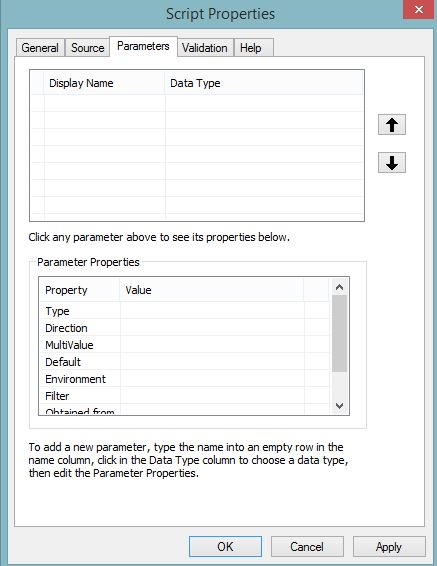and this is how the tool looks like: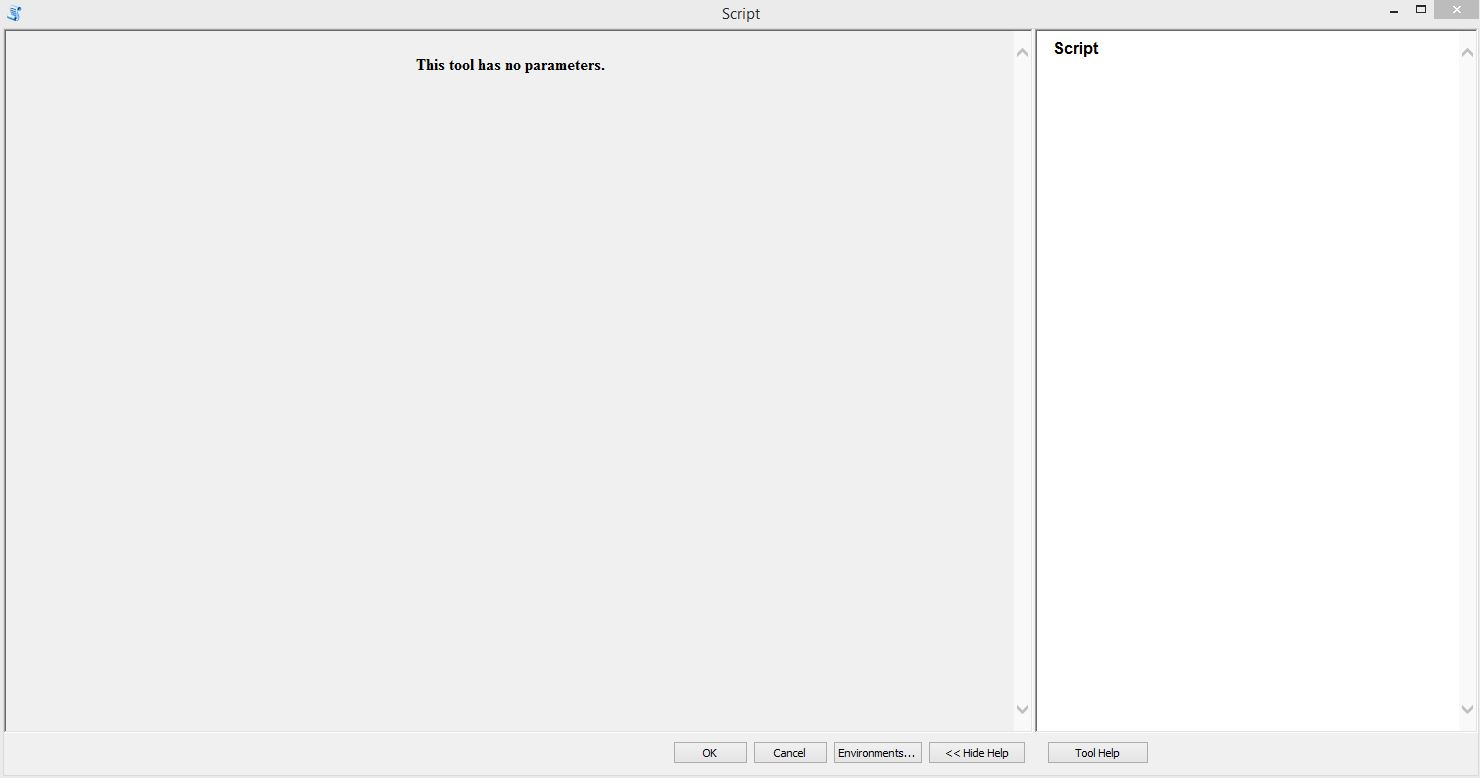byMVP Esteemed Contributor

These lines are the ones that need to be made into script parameters.

``````inputFC = arcpy.GetParameterAsText(0)
outputFC = arcpy.GetParameterAsText(1)
heightfield = arcpy.GetParameterAsText(2) #Must be in the same units as the coordinate system!
azimuth = math.radians(float(arcpy.GetParameterAsText(3))) #Must be in degrees
altitude = math.radians(float(arcpy.GetParameterAsText(4))) #Must be in degrees
‍‍‍‍‍
‍‍‍‍‍‍‍``````

inputFC is an input featureclass

outputFC is the output featureclass

heightfield is the height field in the inputFC (I think... if not, then the outputFC)

azimuth  a number

altitude   dittoNew Contributor II

Thanks Dan Patterson, that's a good start. So now I'm adding the parameters... Defining Input and output was easy. Could you pls tell me how I should define height, Azimuth and altitude? Thanks!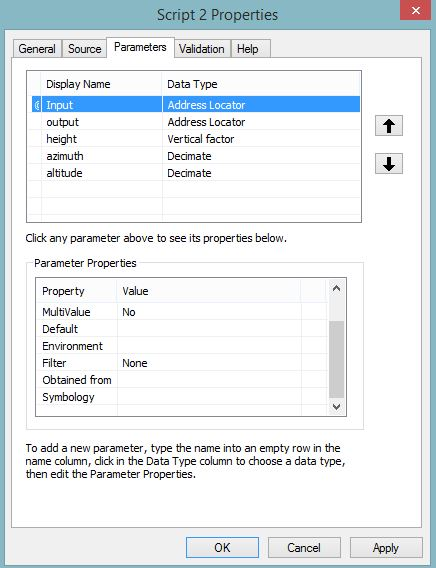byMVP Frequent Contributor

Theory:

In general, parameters are used when running a tool to allow the tool user to pick data sets to be used and to specify where to put the output.  It is not a requirement that you have parameters; but they add flexibility.

If one doesn't want to use parameters, one would have to manually code the script to access the data for the run, to specify where to put the output, specify the workspace, etc.  This is often referred to as "hard coding" the path.  So if the input data was at C:\Data\Modelrun\shadows\20160908, you would have to code that all in the script.  Then repeat for all the other inputs and outputs.  Only then would one convert the script into a tool.

Instead, if one were to set up parameters, when the tool the user would be prompted to browse for the input file, where to export it, etc.  This saves having to recode all the paths every time the file names or file locations change, and then having to rebuild the tool.

Practical use:

Adding parameters will entail learning a little bit about Python.  It's not a big jump, though.

I'm not great at Python, so let me tag some folks who are.

EDIT - I see Dan Patterson  added a reply while I was typing this.

Chris Donohue, GISPNew Contributor II

Thanks Chris Donogue for your comment. Unfortunately I'm not an expert on scripting at all. However I assume the code I which I found on the web can support my research. Still I need to wait to see how I can add it to a toolbox and tun it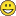byMVP Frequent Contributor

But the actual question here is why the original sun shadow tool, which is built to do this sort of thing, is not working as you expected.

What are the ht values? Is the multipatch output from the tool correctly added to the scene.

Is there something else going on here.New Contributor II

Hi Neil and thanks for your response. Well I have no clue of what the tool shouldn't work. I read other complains on the tool in other forums as well (Here). However the solution someone proposed didn't work for me, as it was not clear what he explained. I have attached a proportion of my data. Feel free to have a look and running the tool. ThanksbyMVP Frequent Contributor

There is no attachment as far as I can see.